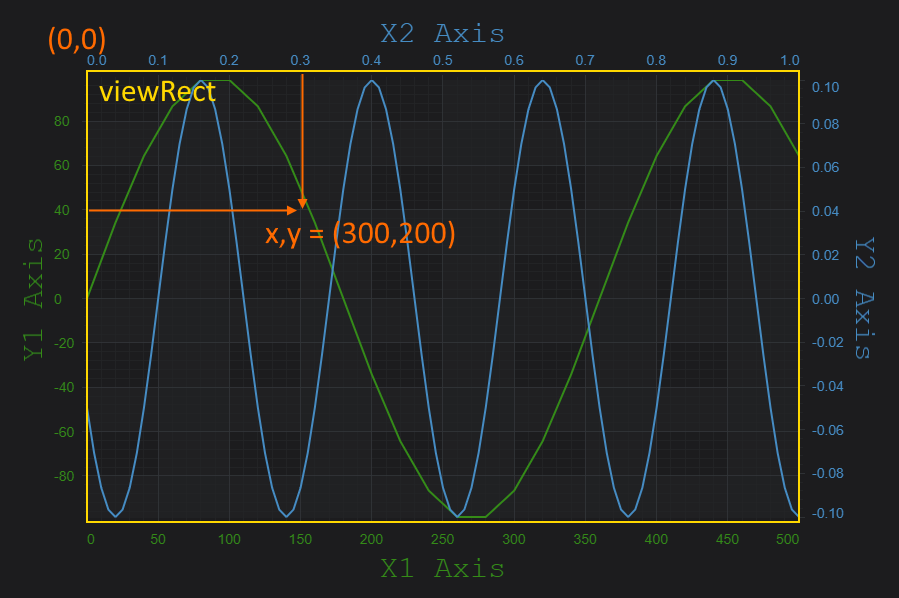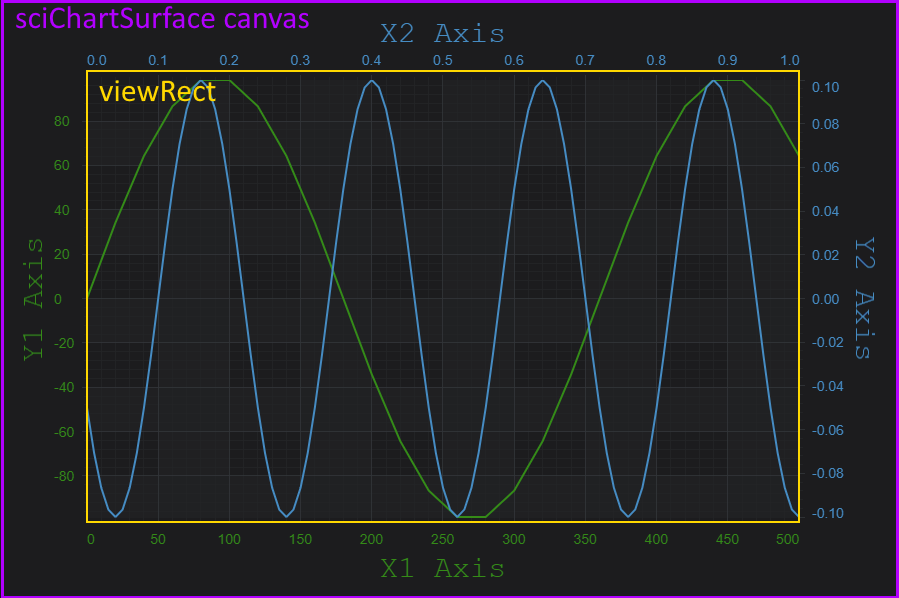# Getting Y coordinate from data

1
0

Hello,

How can i convert my data to Y coordinate value in OhlsSeries?

Version
7.21.0
• You must to post comments
2
0

Hi Artem

You can convert between Data and Pixel coordinates in SciChart.js using this API

Axis APIs – Convert Pixel to Data Coordinates

If you have the data-value for the series, pass it to the YAxis coordinate calculator to do the conversion, like this:

``````const yAxis = sciChartSurface.xAxes.get(0); // Type AxisBase2D
const coordCalc = yAxis.getCurrentCoordinateCalculator(); // Type CoordinateCalculatorBase
// Gets the pixel coordinate relative to viewRect for data-value 1.23 on this axis only
const coord = coordCalc.getCoordinate(1.23);

// Converts a pixel coordinate back to dataValue
const dataValue= coordCalc.getDataValue(coord);

// dataValue should === 1.23
``````

For the x-Axis there is a slightly different approach needed if you are using CategoryAxis. This is covered in the KB article linked above.

Finally, please ensure you understand where coordinates are measured from, also covered in the article above.There is a note in the docs about how to convert between the inner and outer canvas / viewrect in case you need it.Best regards,
Andrew

• You must to post comments
Showing 1 result